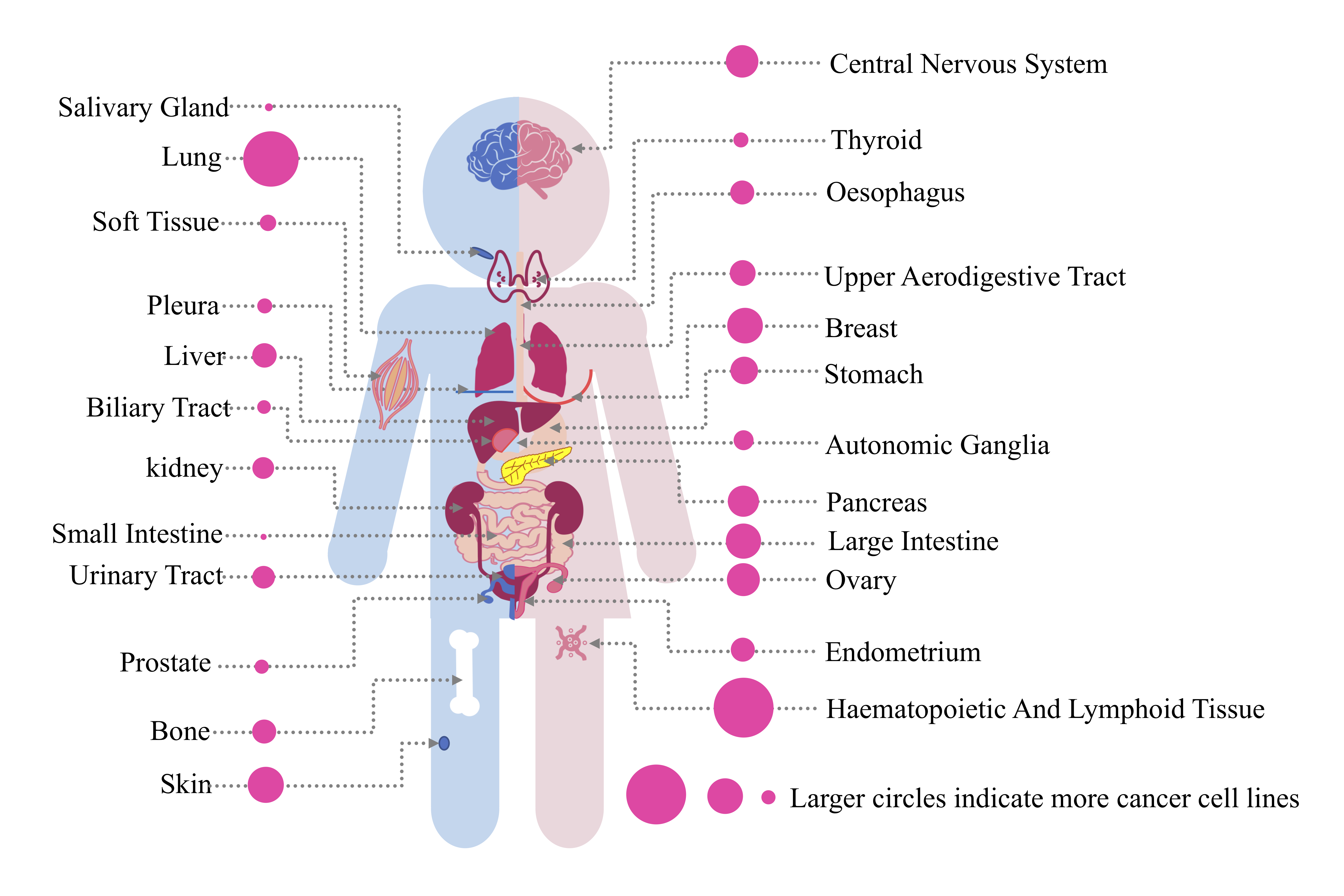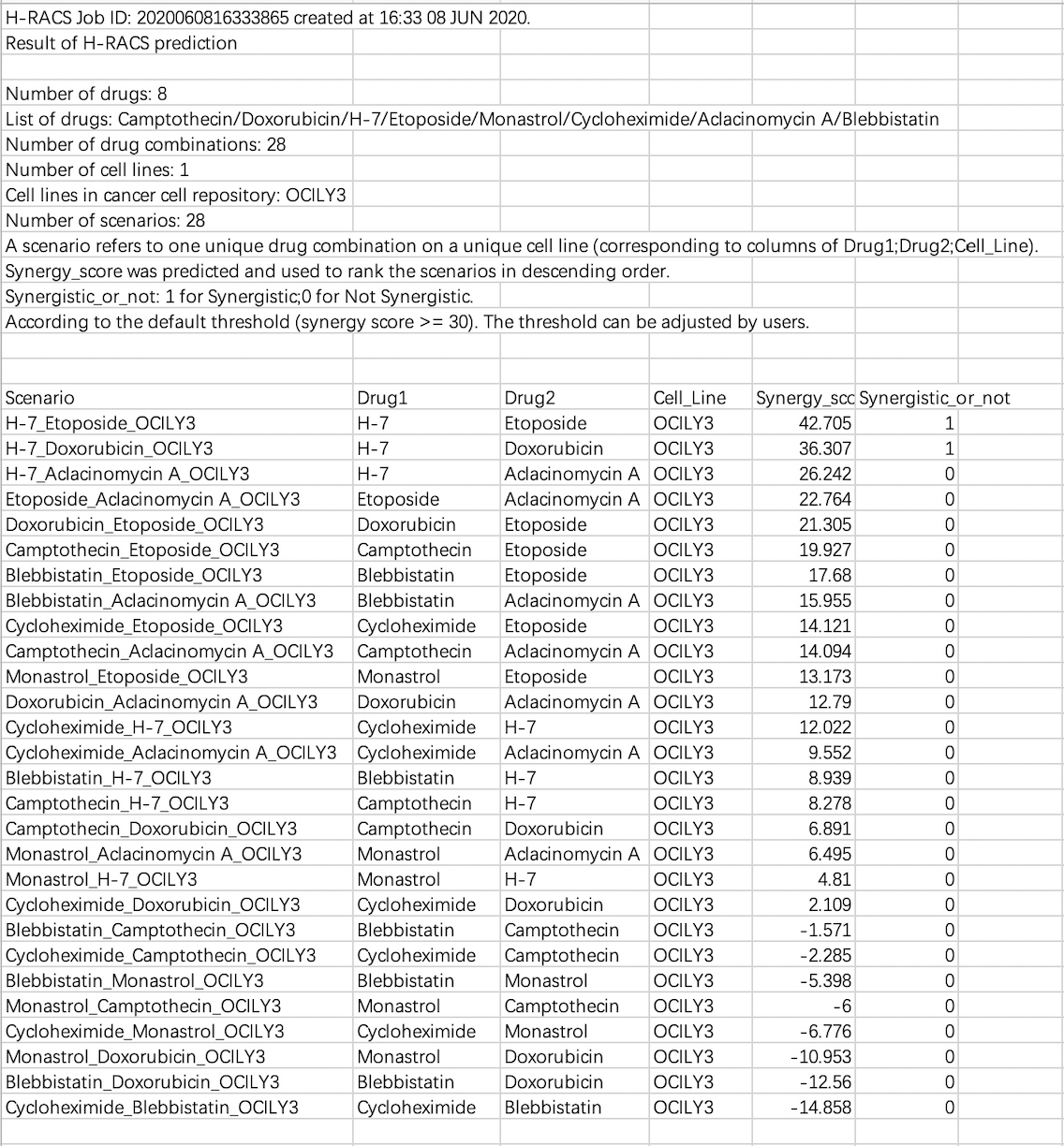A Handy tool to Rank Anti-Cancer Synergistic drugs

# How to use H-RACS

## 1. Submission notice

#### Step 1: Drug information

Drug information file with ".txt" format needs to be uploaded, containing "Drug" (name), "SMILES" (structure) and "Target" (protein), and separated by "Tab" (Table1). Drug targets are tagged with Uniport ID, and connected by commas if more than one.
The standard format file can be downloaded by clicking the button "Drug Example". Batch submission is acceptable.
Please be noted that binary combinations are screened on selected cell lines if multiple drugs are submitted in one file.

#### Table 1. Example of standard format file of drug information.

 Drug SMILES Target Blebbistatin CC1=CC2=C(C=C1)N=C3C(C2=O)(CCN3C4=CC=CC=C4)O Q7Z406 Cycloheximide CC1CC(C(=O)C(C1)C(CC2CC(=O)NC(=O)C2)O)C Q8WTX7,A6NHX0 Camptothecin CCC1(C2=C(COC1=O)C(=O)N3CC4=CC5=CC=CC=C5N=C4C3=C2)O P11387

#### Step2: Cell line

To-be-screened cancer cell lines are submitted via names, connected by comma if multiple. Currently H-RACS can support query on 928 cell lines of 24 tissues (Figure1). If query names are new to H-RACS, message of "the cell line(s) X is(are) not found in Cancer cell lines repository" will be shown for alert.

#### Figure 1. Cancer cell lines repository.After that, you are suggested to provide email address to receive the prediction result. But this is optional. Finally, click the "submit" button to start the computation.

## 2. Example

Step1: Submit drug information

 Drug SMILES Target Doxorubicin CC1C(C(CC(O1)OC2CC(CC3=C(C4=C(C(=C23)O)C(=O)C5=C(C4=O)C=CC=C5OC)O)(C(=O)CO)O)N)O P11388,Q14978 Etoposide CC1OCC2C(O1)C(C(C(O2)OC3C4COC(=O)C4C(C5=CC6=C(C=C35)OCO6)C7=CC(=C(C(=C7)OC)O)OC)O)O P11388,O14733,Q02880 Blebbistatin CC1=CC2=C(C=C1)N=C3C(C2=O)(CCN3C4=CC=CC=C4)O Q7Z406 Cycloheximide CC1CC(C(=O)C(C1)C(CC2CC(=O)NC(=O)C2)O)C Q8WTX7,A6NHX0 Camptothecin CCC1(C2=C(COC1=O)C(=O)N3CC4=CC5=CC=CC=C5N=C4C3=C2)O P11387 H-7 CC12C(C(CC(O1)N3C4=CC=CC=C4C5=C6C(=C7C8=CC=CC=C8N2C7=C53)C(NC6=O)O)NC)OC O15530,O14757,P27448 Monastrol CCOC(=O)C1=C(NC(=S)NC1C2=CC(=CC=C2)O)C P52732 Aclacinomycin A CCC1(CC(C2=C(C1C(=O)OC)C=C3C(=C2O)C(=O)C4=C(C3=O)C=CC=C4O)OC5CC(C(C(O5)C)OC6CC(C(C(O6)C)OC7CCC(=O)C(O7)C)O)N(C)C)O P11388,P11387

Step2: Submit cancer cell lines.
OCI-LY3

## 3. Results interpretation

The result of H-RACS is composed of three parts, including the basic information of submission, synergy score of H-RACS prediction and visualization.

#### 3.1 Information of submission

Basic information on submission is displayed below, and the prediction result can be download from two blue links.#### 3.2 Synergy score result of H-RACS prediction (.csv)This is a result file of the example. As the detail is shown above, among the 28 scenarios derived from 8 drugs on the cell line OCI-LY3, two drug combinations ([H-7, Etoposide], [H-7, Doxorubicin]) were predicted as the synergistic drug combinations on cell line OCI-LY3.

#### 3.3 Visualization of the prediction result (.svg)

To make the result clearer, we visualize the result as below. the redder as a drug combination's synergy score shown, the more synergistic this drug combination is predicted on this given cell line.## 4. Browser compatibility

 OS Version Chrome FireFox Microsoft Edge Safari Linux 4.13.0(ubuntu17.10) 71.0.3578.98 61.0.1 n/a n/a MacOS Catalina 10.15 78.0.3904.108 70.0 n/a 13.0.2 Windows 10.0.17134 78.0.3904.108 70.0.1 42.17134.1.0 n/a